Home > CCA2 > Chapter 12 > Lesson 12.1.1 > Problem12-9

12-9.
1. Given the function f(x) = 2 +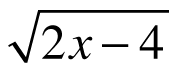, find: Homework Help ✎

1. The domain and range.

2. The inverse equation.

3. The domain and range of the inverse.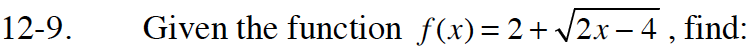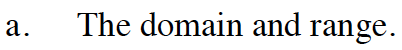Sketch a graph. What is the smallest x-value you can use before the number under the radical sign becomes negative?
What is the smallest y-value that can result from this equation?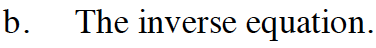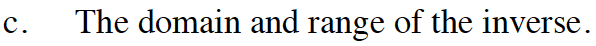Remember that in an inverse, x and y are interchanged. Reverse the domain and range you found in part (a).